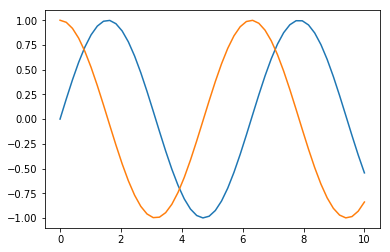# Predefined output

When the option predefinedOutput has been set to true and the next element after the pre tag has data-output set to true, the html content of the next element will be rendered as a preview before execution.

## Predefined text

A cell with the text 'Hello!' set as predefined output.

```    print("Hello!")
```
Hello!

## Predefined image

A cell with an image set as predefined output.

```%matplotlib inline
import numpy as np
import matplotlib.pyplot as plt
x = np.linspace(0,10)
plt.plot(x, np.sin(x))
plt.plot(x, np.cos(x))
```